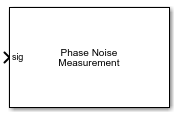Main Content

# Phase Noise Measurement

Compute phase noise at specific frequency offset vectors

• Library:
• Mixed-Signal Blockset / PLL / Measurements & Testbenches

•## Description

The Phase Noise Measurement block measures and plots the phase noise profile for a time domain signal. It also displays the phase noise values for the specified frequency offset vector on the icon of the block.

## Ports

### Input

expand all

Input time domain signal to the Phase Noise Measurement block, specified as a scalar.

Data Types: `double`

## Parameters

expand all

Smallest positive frequency that can be resolved, specified as a real positive scalar. The Resolution bandwidth (Hz) is used to determine window length for spectral analysis using the Welch method. For more information, see Spectrum Estimation — Welch's Method.

In general, Resolution bandwidth (Hz) should be less than or equal to the lowest offset frequency value.

#### Programmatic Use

• Use `get_param(gcb,'ResBandwidth')` to view the current value of Resolution bandwidth (Hz).

• Use `set_param(gcb,'ResBandwidth',value)` to set Resolution bandwidth (Hz) to a specific value.

Number of spectral averages, specified as a positive integer scalar. The `dsp.SpectrumEstimator` System object™ used by the Phase Noise Measurement block computes the current power spectrum or power density spectrum estimate by averaging over the number specified by No. of spectral averages.

#### Programmatic Use

• Use `get_param(gcb,'SpectralAverages')` to view the current value of No. of spectral averages.

• Use `set_param(gcb,'SpectralAverages',value)` to set No. of spectral averages to a specific value.

Delays measurement analysis by the specified amount of time to avoid corruption by transients, specified as a real nonnegative scalar in s.

#### Programmatic Use

• Use `get_param(gcb,'HoldOffTime')` to view the current value of Hold off time (s).

• Use `set_param(gcb,'HoldOffTime',value)` to set Hold off time (s) to a specific value.

Frequency points relative to fundamental frequency where phase noise is calculated, specified as a real valued vector in Hz.

#### Programmatic Use

• Use `get_param(gcb,'PhaseNoiseFreqOffset')` to view the current value of Frequency offset vector (Hz).

• Use `set_param(gcb,'PhaseNoiseFreqOffset',value)` to set Frequency offset vector (Hz) to a specific value.

Specify the target phase noise vector for a given frequency offset vector. By default, this option is deselected

Target phase noise profile for given frequency offset vector, specified as a real valued vector in dBc/Hz. Phase noise vector (dBc/Hz) is the phase noise power in a 1 Hz bandwidth centered at the specified frequency offsets relative to the carrier

#### Dependencies

To enable this parameter, select the Specify target parameter.

#### Programmatic Use

• Use `get_param(gcb,'TargetPhaseNoise')` to view the current value of Phase noise vector (dBc/Hz).

• Use `set_param(gcb,'TargetPhaseNoise',value)` to set Phase noise vector (dBc/Hz) to a specific value.

Click to set the Recommended min. simulation stop time (s) reported by the Phase Noise Measurement block as the model stop time.

Click to plot the measured phase noise profile of the input signal. Target phase noise profile is also overlayed if the Specify target option is selected. You can plot the phase noise profile any time during simulation.

Click to export measurement results to an excel spreadsheet (XLS) or as comma-separated values (CSV) after the simulation is complete.

## Algorithms

The Phase Noise Measurement block uses the zero crossing points of a signal to measure the phase noise. From the zero crossing points, the phase error (φ)is extracted both at the rising and falling edge of the signal. The captured phase error is used to generate a periodic signal (sin(φ)). This periodic signal is interpolated using a fixed time step proportional to the maximum phase noise frequency offset. The power density of the modified phase noise signal, using the `dsp.SpectrumEstimator` System object, directly provides the phase noise profile of the signal of interest.

Introduced in R2019a

## SupportGet trial now